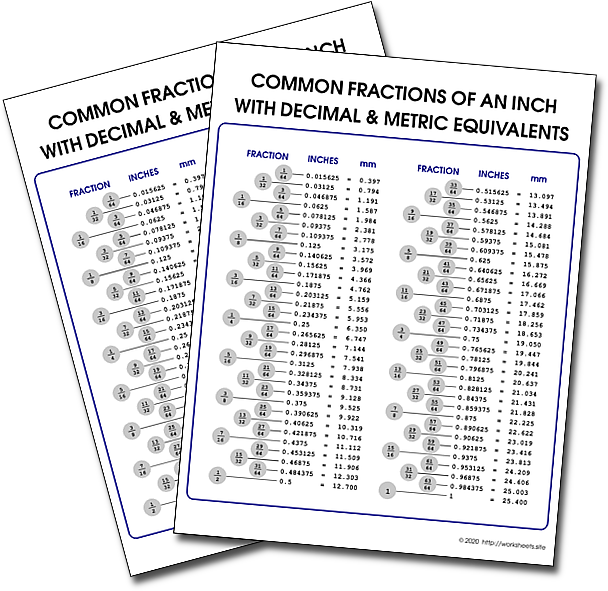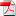# Fractions to Decimal Chart PDF

Common fractions of an inch with decimal and metric equivalents. Decimal Equivalents of Fractions. Printable Decimal to Fraction convertion chart. Fraction-Decimal Converter. Fraction Inch to Metric conversion. Drill Sizes. mm to inch.Use the password worksheets.site to open the PDF file.

## 1. How to Convert Fractions to Decimals

You can convert all fractions to decimals. The decimal forms of rational numbers either end or repeat a pattern. To convert fractions to decimals you just divide the top by the bottom — divide the numerator by the denominator — and if the division doesn´t come out evenly, you can stop after a certain number of decimal places and round off.

To convert fractions to decimals:

• If the fraction is a mixed number, change it to an improper fraction.
• Divide the numerator by the denominator.
• If the division doesn´t come out evenly, round the decimal off.

## 2. How to Convert Decimals to Fractions

Any decimal can be converted to a fraction by following a few steps. We explain how to convert both terminating decimals and repeating decimals to fractions.

### 2.1. Converting a Terminating Decimal to a Fraction

A terminating decimal is any decimal that has a finite other of digits. In other words, it has an end. Examples include .5, .234, .864721, etc. Terminating decimals are the most common decimals you´ll see and, fortunately, they are also the easiest to convert to fractions.

#### 2.1.1. Step 1

Write the decimal divided by one.

For example, say you´re given the decimal .55. Your first step is to write out the decimal so it looks like .55/1.

#### 2.1.2. Step 2

Next, you want to multiply both the top and bottom of your new fraction by 10 for every digit to the left of the decimal point.

In our example, .55 has two digits after the decimal point, so we´ll want to multiply the entire fraction by 10 x 10, or 100. Multiplying the fraction by 100/100 gives us 55/100.

#### 2.1.3. Step 3

The final step is reducing the fraction to its simplest form. The simplest form of the fraction is when the top and bottom of the fraction are the smallest whole numbers they can be. For example, the fraction 3/9 isn´t in its simplest form because it can still be reduced down to 1/3 by dividing both the top and bottom of the fraction by 3.

The fraction 55/100 can be reduced by dividing both the top and bottom of the fraction by 5, giving us 11/20. 11 is a prime number and can´t be divided any more, so we know this is the fraction in its simplest form.

The decimal 0.55 is equal to the fraction 11/20.

### 2.2. Converting a Repeating Decimal to a Fraction

A repeating decimal is one that has no end. Since you can´t keep writing or typing the decimal out forever, they are often written as a string of digits rounded off (.666666667) or with a bar above the repeating digit(s) $\overline{)\left(.6\right)}$.

For our example, we´ll convert .6667 to a fraction.

The decimal .6667 is equal to $\overline{)\left(.6\right)}$, .666666667, .667, etc. They´re all just different ways to show that the decimal is actually a string of 6´s that goes on forever.

#### 2.2.1. Step 1

Let x equal the repeating decimal you´re trying to convert, and identify the repeating digit(s).

So x=.6667

6 is the repeating digit, and the end of the decimal has been rounded up.

#### 2.2.2. Step 2

Multiply by whatever value of 10 you need to get the repeating digit(s) on the left side of the decimal.

For .6667, we know that 6 is the repeating digit. We want that six on the left side of the decimal, which means moving the decimal place over one spot. So we multiply both sides of the equation by (10 x 1) or 10.

10x = 6.667

Note: You only want one “set” of repeating digit(s) on the left side of the decimal. In this example, with 6 as the repeating digit, you only want one 6 on the left of the decimal. If the decimal was 0.58585858, you´d only want one set of “58” on the left side. If it helps, you can picture all repeating decimals with the infinity bar over them, so .6667 would be $\overline{)\left(.6\right)}$.

#### 2.2.3. Step 3

Next we want to get an equation where the repeating digit is just to the right of the decimal.

Looking at x = .6667, we can see that the repeating digit (6) is already just to the right of the decimal, so we don´t need to do any multiplication. We´ll keep this equation as x = .6667

#### 2.2.4. Step 4

Now we need to solve for x using our two equations, x = .667 and 10x = 6.667.

10x - x = 6.667 - .667

9x = 6

x = 6/9

x = 2/3

## 3. Printable Chart

Walk into any metal fabrication shop and you will see some version of our chart on the wall. This version can be printed in any size because it is not a raster image, instead it's a high resolution PDF. These popular charts are frequently used in woodwork shops too, and can used in the classroom to demostrate conversions between different units of length. Use the password worksheets.site to open the file.